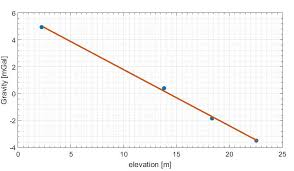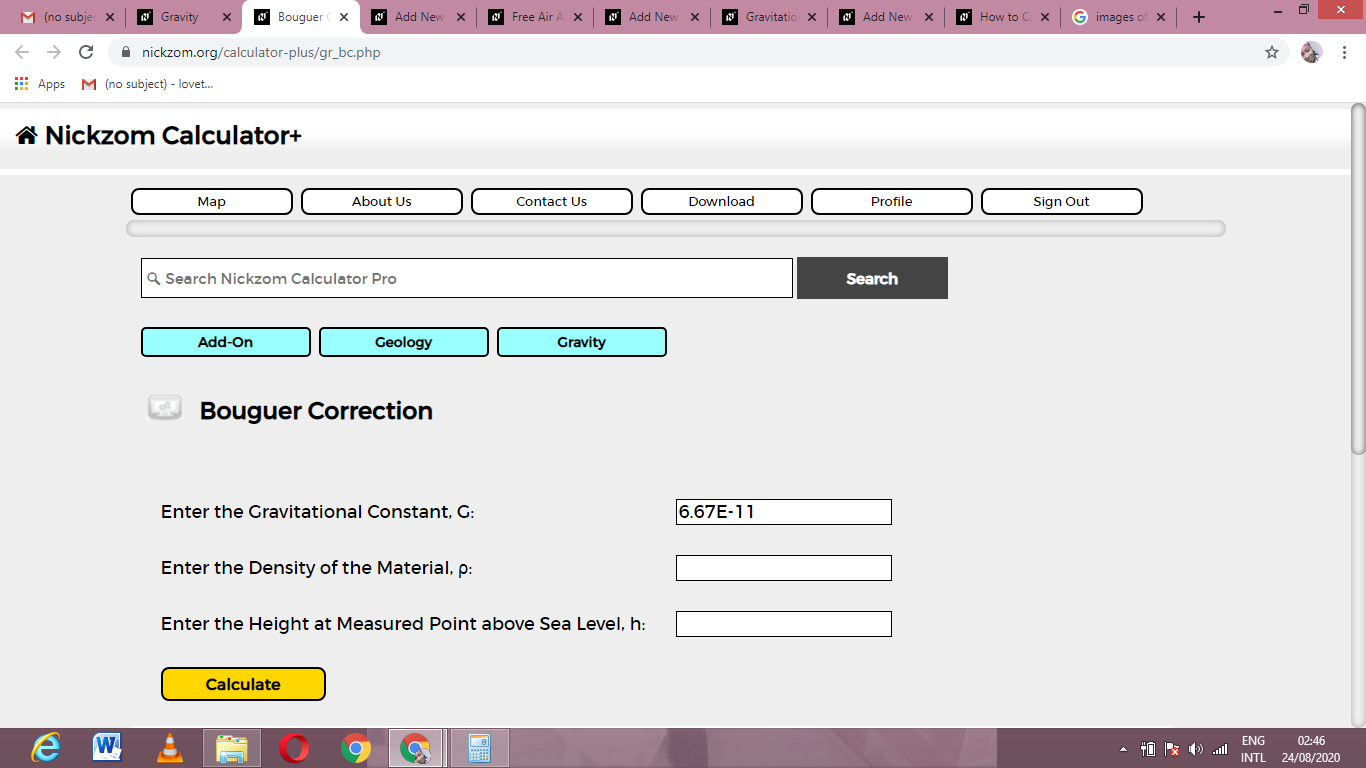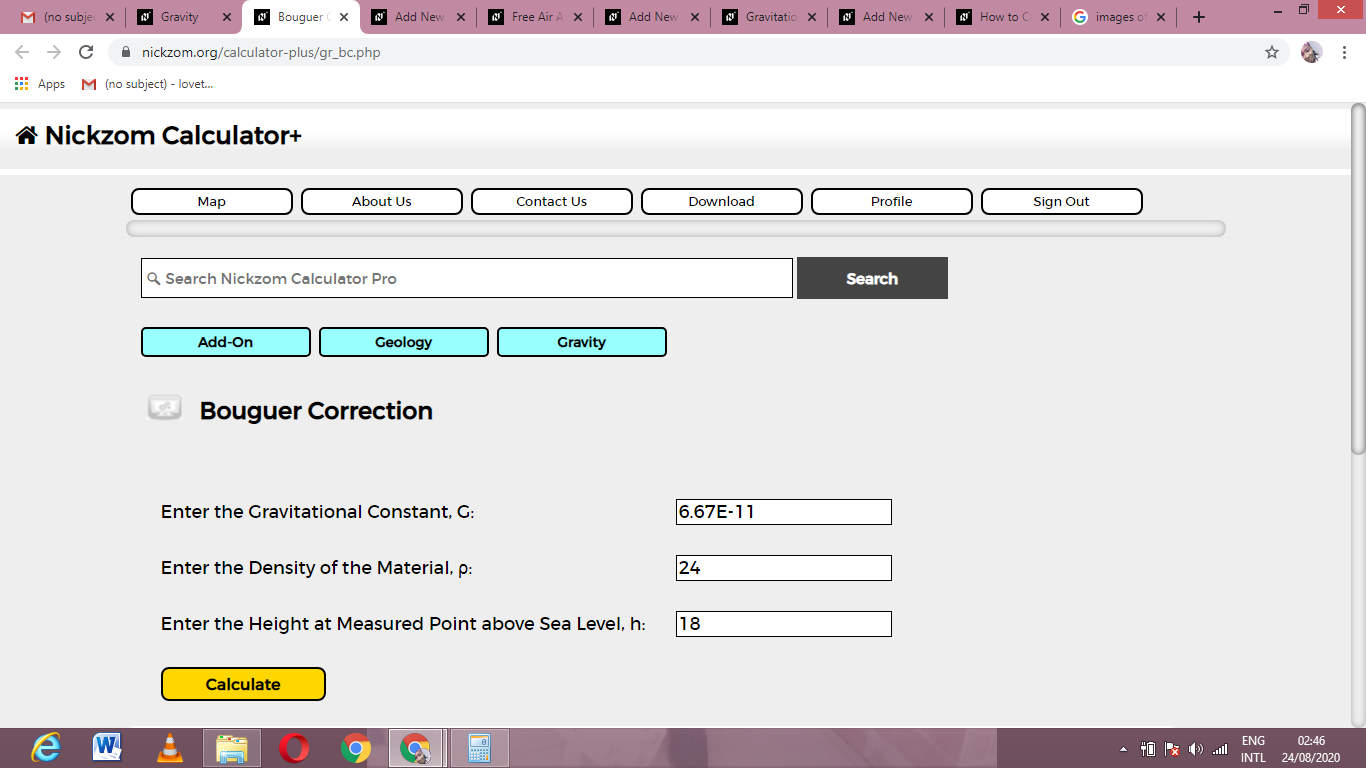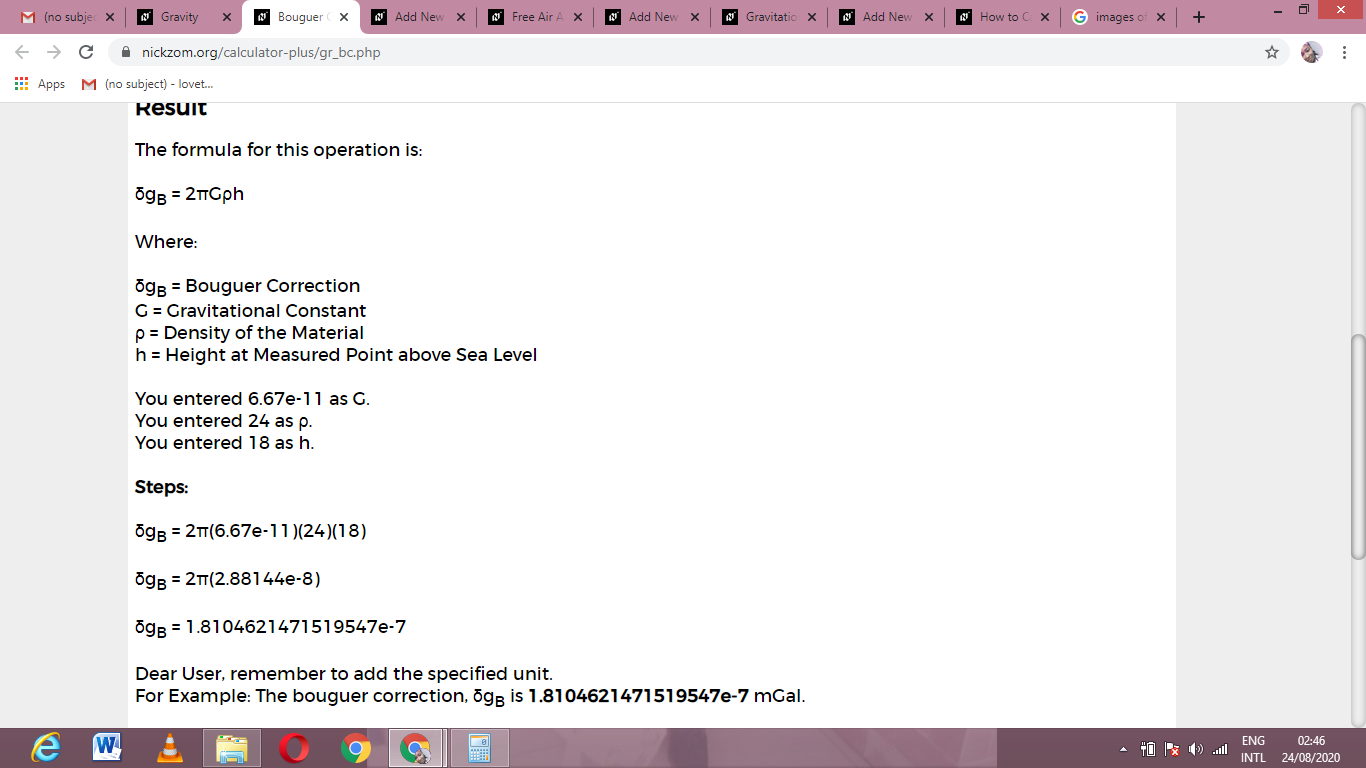# How to Calculate and Solve for Bouguer Correction | GravityThe image above represents bourger correction.

To compute for bourger correction, three essential parameter are needed and these parameters are Gravitational Constant (G), Density of the Material (ρ) and Height at Measured Point above Sea Level (h).

The formula for calculating bourger correction:

δgB = 2πGρh

Where:

δgB = Bouguer Correction
G = Gravitational Constant
ρ = Density of the Material
h = Height at Measured Point above Sea Level

Let’s solve an example;
Find the bourger correction when the gravitational constant is 6.67E-11, the density of the material is 24 and the height at measured point above sea level is 18.

This implies that;

G = Gravitational Constant = 6.67E-11
ρ = Density of the Material = 24
h = Height at Measured Point above Sea Level = 18

δgB = 2πGρh
δgB = 2π(6.67e-11)(24)(18)
δgB = 2π(2.88144e-8)
δgB = 1.81e-7

Therefore, the bourger correction is 1.81e-7 mGal.

Calculating the Density of the Material when the Bourger Correction, the Gravitational Constant and the Heigth at Measured Point above Sea Level is Given.

ρ = δgB / 2πGh

Where;

ρ = Density of the Material
δgB = Bouguer Correction
G = Gravitational Constant
h = Height at Measured Point above Sea Level

Let’s solve an example;
Find the density of the material when the bourger correction is 22, the gravitational constant is 6.67E-11 and the height at measured point above sea level is 15.

This implies that;

δgB = Bouguer Correction = 22
G = Gravitational Constant = 6.67E-11
h = Height at Measured Point above Sea Level = 15

ρ = δgB / 2πGh
ρ = 22 / 2π (6.67e-11)(10)
ρ = 22 / 2π x 6.67e+11
ρ = 22 / 4188760000000
ρ = 5.25e-12

Therefore, the density of the material is 5.25e-12.

Calculating the Height at Measured Point above Sea Level when the Bourger Correction, the Gravitational Constant and the Density of the Material is Given.

h = δgB / 2πGρ

Where;

h = Height at Measured Point above Sea Level
δgB = Bouguer Correction
G = Gravitational Constant
ρ = Density of the Material

Let’s solve an example;
Find the height at measured point above sea level when the bourger correction is 32, the gravitational constant is 6.67E-11 and the density of the material is 10.

This implies that;

δgB = Bouguer Correction = 32
G = Gravitational Constant = 6.67E-11
ρ = Density of the Material = 10

h = δgB / 2πGρ
h = 32 / 2π (6.67e-11)(10)
h = 32 / 4188760000000
h = 7.639e-12

Therefore, the height at measured point above sea level is 7.639e-12.

Nickzom Calculator – The Calculator Encyclopedia is capable of calculating the bourger correction.

To get the answer and workings of the bourger correction using the Nickzom Calculator – The Calculator Encyclopedia. First, you need to obtain the app.

You can get this app via any of these means:

To get access to the professional version via web, you need to register and subscribe for NGN 2,000 per annum to have utter access to all functionalities.
You can also try the demo version via https://www.nickzom.org/calculator

Apple (Paid) – https://itunes.apple.com/us/app/nickzom-calculator/id1331162702?mt=8
Once, you have obtained the calculator encyclopedia app, proceed to the Calculator Map, then click on Geology under Add-on.Now, Click on Gravity under GeologyNow, Click on Bourger Correction under GravityThe screenshot below displays the page or activity to enter your values, to get the answer for the bourger correction according to the respective parameters which are the Gravitational Constant (G), Density of the Material (ρ) and Height at Measured Point above Sea Level (h).Now, enter the values appropriately and accordingly for the parameters as required by the Gravitational Constant (G) is 6.67e-11, Density of the Material (ρ) is 24 and Height at Measured Point above Sea Level (h) is 18.Finally, Click on CalculateAs you can see from the screenshot above, Nickzom Calculator– The Calculator Encyclopedia solves for the bourger correction and presents the formula, workings and steps too.